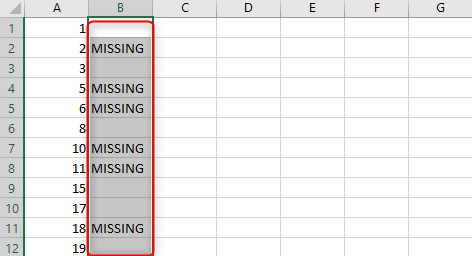# Excel How to Find Missing Numbers in a Sequence

Sometimes we have a long list of sequence numbers to mark items or commodities, like having check numbers in bank statements. Most people scroll through the whole list to locate missing numbers. It is tiresome and consumes a lot of time. Using excel 2007, 2010, and 2013, it has tricks to get the missing number in a sequence. There are many ways to do this. I will show you two of them and choose which one suits you.

• IF Formula is based on that numbers in sequence increase by one. If the number is not less than one, we have one missing.
• Array formula, the Formula not identify missing numbers in the sequence but also list the missing number identified.

## Identify Missing numbers in sequence using IF Formula

Here is a list of my number; I just picked random numbers to let's work it out and see.1. Look for a blank cell and write this Formula =IF(A3-A2=1, "," MISSING"); for me, I will use B2 cell. Hit enter. If there is a missing number, the formula output will be the word missing inactive cell within the column you wrote the Formula. For cells with no missing numbers, it will return nothing.2. Select B2 and drag it down to select the number of cells you want the Formula to be or identify the missing numbers. The Formula indicates the missing numbers with the word MISSING in cells of column B.3. Fill in the gaps for the next value. That's manual, we have a Formula for that, but we will talk about it later.

## Using array formula to identify missing numbers in a sequence

The Formula eases the work as the Array formula identifies and lists the missing values.

1. In the adjacent cell, write the array formula, which is =SMALL(IF(MATCH(ROW(A\$1:A\$20), A\$1:A\$20)), ROW(A\$1:A\$30)), ROW(A1)). After writing the Formula, don't hit enter; press CTRL + SHIFT + ENTER at the same time. And here is what you will see.A\$1:A\$30 is the range of cells you want to identify missing numbers.

It shows one of the missing numbers; to get the rest of the values, we git to step 2.

2. Select cell B1 and drag it down from its corner to fill the whole column. It will indicate the missing numbers, as shown below.4,7,9,12,13,14,16, and 20 are missing; even if you follow manually, you will get the same outcome. You won't learn it the first time; you need to practice for a long time to be an expert in this.

3. Fill in the numbers.

There are other more complex Formulas than this two, for example, the use of kutool excel. It is a powerful feature that can be installed on excel? It solves a complex problems like having a list with AA-1001, AA-1002-BBs. Let's say it solve large and complex numbers.

To use this tool, we need to download and install kutool for excel. In our next tutorial, we will be using this tool.